### Output Data Set

PROC PRINQUAL produces an output data set by default. By specifying the OUT=, APPROXIMATIONS, SCORES, REPLACE, and CORRELATIONS options in the PROC PRINQUAL statement, you can name this data set and control its contents.

By default, the procedure creates an output data set that contains variables with _TYPE_=’SCORE’. These observations contain original variables, transformed variables, components, or data approximations. If you specify the CORRELATIONS option in the PROC PRINQUAL statement, the data set also contains observations with _TYPE_=’CORR’; these observations contain correlations or component structure information.

#### Structure and Content

The output data set can have 16 different forms, depending on the specified combinations of the REPLACE, SCORES, APPROXIMATIONS, and CORRELATIONS options. You can specify any combination of these options. To illustrate, assume that the data matrix consists of N observations and m variables, and n components are computed. Then define the following: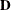the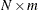matrix of original data with variable names that correspond to the names of the variables in the input data set. However, when you use the OPSCORE transformation on character variables, those variables are replaced by numeric variables that contain category numbers.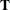thematrix of transformed data with variable names constructed from the value of the TPREFIX= option (if you do not specify the REPLACE option) and the names of the variables in the input data set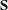the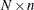matrix of component scores with variable names constructed from the value of the PREFIX= option and integers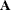thematrix of data approximations with variable names constructed from the value of the APREFIX= option and the names of the variables in the input data set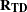thematrix of correlations between the transformed variables and the original variables with variable names that correspond to the names of the variables in the input data set. When missing values exist, casewise deletion is used to compute the correlations.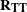thematrix of correlations among the transformed variables with the variable names constructed from the value of the TPREFIX= option (if you do not specify the REPLACE option) and the names of the variables in the input data set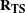thematrix of correlations between the transformed variables and the principal component scores (component structure matrix) with variable names constructed from the value of the PREFIX= option and integers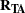thematrix of correlations between the transformed variables and the variable approximations with variable names constructed from the value of the APREFIX= option and the names of the variables in the input data set

To create a data set Work.A that contains all information, specify the following options in the PROC PRINQUAL statement:

proc prinqual scores approximations correlations out=a;


Also use a TRANSFORM statement appropriate for your data. Then the Work.A data set contains the following:

 D T S A R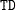R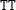R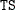R
To eliminate the bottom partitions that contain the correlations and component structure, do not specify the CORRELATIONS option. For example, use the following PROC PRINQUAL statement with an appropriate TRANSFORM statement:

proc prinqual scores approximations out=a;


Then the Work.A data set contains the following:

   D T S A


Suppose you use the following PROC PRINQUAL statement (with an appropriate TRANSFORM statement):

proc prinqual out=a;


This creates a data set Work.A of the following form:

   D T


To output transformed data and component scores only, specify the following options in the PROC PRINQUAL statement:

proc prinqual replace scores out=a;


Then the Work.A data set contains the following:

   T S


#### _TYPE_ and _NAME_ Variables

In addition to the preceding information, the output data set contains two character variables, the variable _TYPE_ (length 8) and the variable _NAME_ (length 32).

The _TYPE_ variable has the value ’SCORE’ if the observation contains variables, transformed variables, components, or data approximations; the _TYPE_ variable has the value ’CORR’ if the observation contains correlations or component structure.

By default, the _NAME_ variable has values ’ROW1’, ’ROW2’, and so on, for the observations with _TYPE_=’SCORE’. If you use an ID statement, the variable _NAME_ contains the formatted ID variable for SCORES observations. The values of the variable _NAME_ for observations with _TYPE_=’CORR’ are the names of the transformed variables.

Certain procedures, such as PROC PRINCOMP, which can use the PROC PRINQUAL output data set, issue a warning that the PROC PRINQUAL data set contains _NAME_ and _TYPE_ variables but is not a TYPE=CORR data set. You can ignore this warning.

#### Variable Names

The TPREFIX=, APREFIX=, and PREFIX= options specify prefixes for the transformed and approximation variable names and for principal component score variables, respectively. PROC PRINQUAL constructs transformed and approximation variable names from a prefix and the first characters of the original variable name. The number of characters in the prefix plus the number of characters in the original variable name (including the final digits, if any) required to uniquely designate the new variables should not exceed 32. For example, if the APREFIX= parameter that you specify is one character, PROC PRINQUAL adds the first 31 characters of the original variable name; if your prefix is four characters, only the first 28 characters of the original variable name are added.

#### Effect of the TSTANDARD= and COVARIANCE Options

The values in the output data set are affected by the TSTANDARD= and COVARIANCE options. If you specify TSTANDARD=NOMISS, the NOMISS standardization is performed on the transformed data after the iterations have been completed, but before the output data set is created. The new means and variances are used in creating the output data set. Then, if you do not specify the COVARIANCE option, the data are transformed to mean zero and variance one. The principal component scores and data approximations are computed from the resulting matrix. The data are then linearly transformed to have the mean and variance specified by the TSTANDARD= option. The data approximations are transformed so that the means within each pair of a transformed variable and its approximation are the same. The ratio of the variance of a variable approximation to the variance of the corresponding transformed variable equals the proportion of the variance of the variable that is accounted for by the components model.

If you specify the COVARIANCE option and do not specify TSTANDARD=Z, you can input the transformed data to PROC PRINCOMP, again specifying the COVARIANCE option, to perform a components analysis of the results of PROC PRINQUAL. Similarly, if you do not specify the COVARIANCE option with PROC PRINQUAL and you input the transformed data to PROC PRINCOMP without the COVARIANCE option, you receive the same report. However, some combinations of PROC PRINQUAL options, such as COVARIANCE and TSTANDARD=Z, while valid, produce approximations and scores that cannot be reproduced by PROC PRINCOMP.

The component scores in the output data set are computed from the correlations among the transformed variables, or from the covariances if you specified the COVARIANCE option. The component scores are computed after the TSTANDARD=NOMISS transformation, if specified. The means of the component scores in the output data set are always zero. The variances equal the corresponding eigenvalues, unless you specify the STANDARD option; then the variances are set to one.